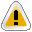﻿ Getting Started Tutorials - MechDesigner > Tutorial 13: Forces: Introduction

# Tutorial 13: Forces: Introduction

Navigation:  Getting Started Tutorials - MechDesigner >

# Tutorial 13: Forces: Introduction

## Force Analysis

The objective of this tutorial is to understand and use the:

Forces Toolbar

Force Vectors

Configure Power Source dialog-boxGravitational Force acts vertically down, () in the direction of Model's 'Global – Y-axis'. The 'Front View' (Short-Cut: F3 key) Therefore, when the force results will be important, you must start the design on the plane that represents the orientation of the actual machine. If, later you want to move a mechanism to a different plane, you must save the Model as a Library File, then select a different Plane to open the Library File.